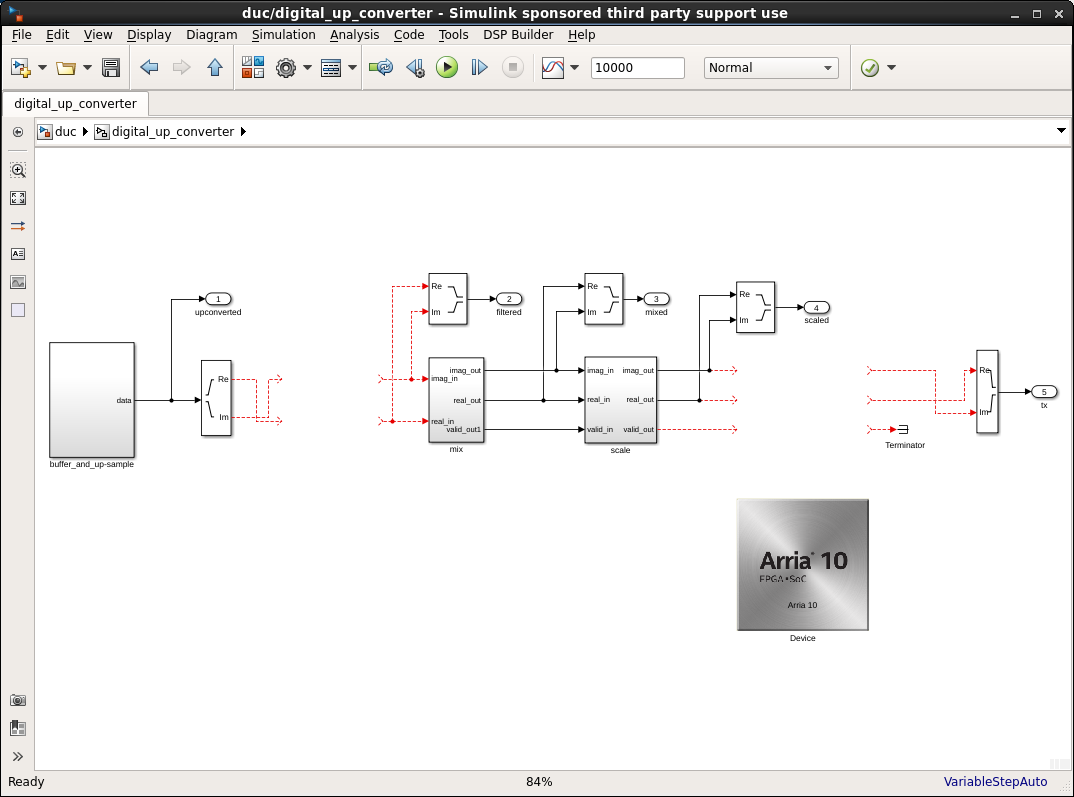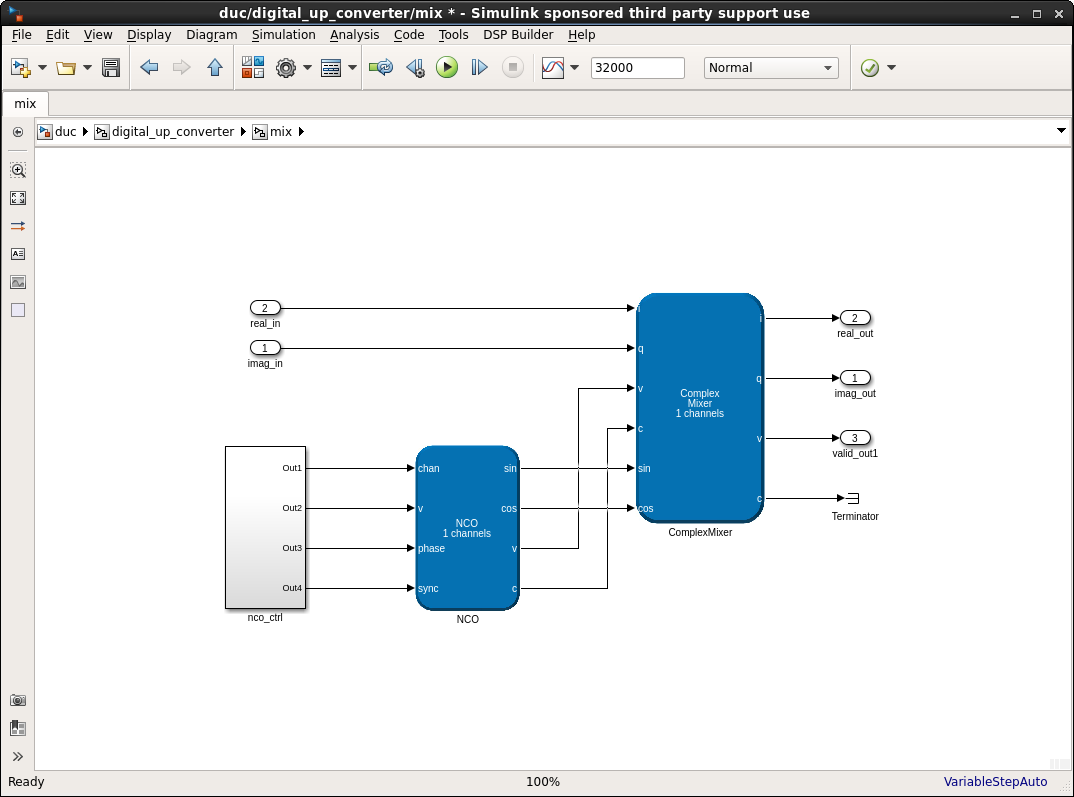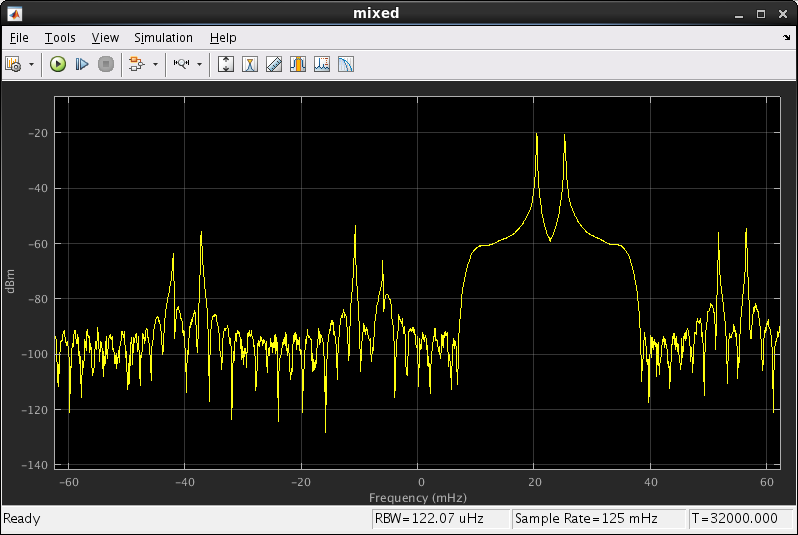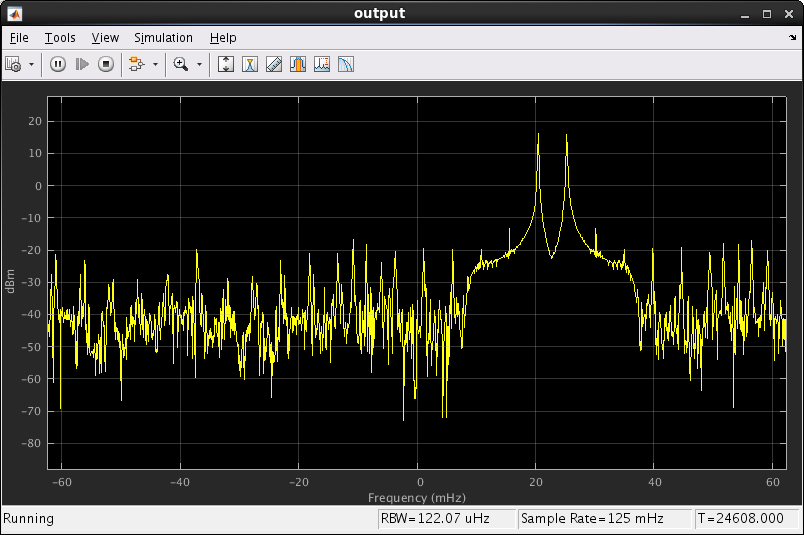ID 683337
Date 6/26/2023
Public

Give Feedback

## 7.9. DSP Builder HDL Import Design Example

This digital up-converter resamples 20 MSPS complex base-band data to 80 MHz intermediate frequency, mixes it to center on +25 MHz, and applies some simple digital predistortion (DPD). This design example takes FIR and DPD VHDL components to create a complete up-conversion chain by importing existing IP and adding the up-conversion, mixer and pre-DPD scaling.

The digital upconverter includes: input memory, upconverter, FIR filter, scaler, mixer and digital predistortion (DPD).

 hdl_import_duc.mdl The DSP Builder design. hdl_import_duc_params.xml The design's parameter file. hdl_import_calc_fir_coefs.m A script to generate the FIR coefficients using MATLAB's cfirpm function. DSP Builder prints the coefficients to MATLAB's Command Window and you can copy and paste them into coefficients.vhd. calc_dpd_coefs.m A script to generate the DPD coefficients using a simple polynomial model of a power amplifier. DSP Builder prints the coefficients MATLAB's Command Window and you can copy and paste them into lut_dpd.vhd. to_import This directory contains 12 VHDL source files.

### VHDL Components

The design example includes a complex FIR filter in VHDL optimized for Intel Stratix 10 devices. This FIR filter has one valid data sample every eight clock cycles.

See Designing Filters for High Performance.

The simple LUT-based DPD is initialized with a third-order polynomial.

### Top-Level Design

The top-level design contains the device-level subsystem and five downsample and spectrum analyzer blocks from MathWork's DSP System Toolbox. These blocks show the spectral output from the various stages of the up-conversion chain.

Figure 60. Top-Level Design### Digital Up Converter

The digital_up_converter subsystem is the device-level subsystem. It contains all of the design's DSP Builder-based components and two gaps for HDL Import blocks.

Figure 61. Digital Upconverter### Buffer and Upsample

This scheduled subsystem contains two SharedMem blocks, which contain the 20 MSPS baseband source: one for the real part of the signal and one for the imaginary part. You can write to the blocks via the bus or use the preloaded tones.

The read_counter block drives the upconversion. It counts modulo 32 because it upsamples the 20 MSPS baseband by 4 to 80 MSPS and then holds each sample for 8 clock cycles at a clock rate of 640 MHz. The FIR filter accepts one sample every eight cycles. By holding the samples, the FIR does not need synchronization logic.

Figure 62. Buffer and Up-Sample### Mixer

This single-channel mixer consists of NCO and ComplexMixer IP blocks and a scheduled subsystem for controlling the NCO. The control subsystem asserts the valid signal once every eight cycles. The NCO generates a 16 MHz complex tone, which the ComplexMixer uses to mix the filtered signal.

Figure 63. Mixer### Scale

The scale scheduled subsystem scales the data so that it fits within the DPD's range of operation by bit-shifting from the mixer's output. You can use the optional multiplier for increasing the signal level if bit-shifting is insufficient.

Figure 64. Scale### FIR Coefficients

The FIR coefficients are defined in coefficients.vhd.

The coefficients are calculated in hdl_import_calc_fir_coefs.m. This script uses MATLAB’s cfirpm command to create complex coefficients.

### DPD

The file lut_dpd.vhd contains the DPD for this design example. The DPD consists of an address generator that indexes a LUT. The output of the LUT is then multiplied with the complex input data. The LUT contents are calculated in hdl_import_calc_dpd_coefs.m. This script uses a simple, real-numbered, third-order model of an amplifier to calculate predistortion coefficients. DSP Builder uses these coefficients to calculate the LUT contents.

Figure 65. Simulation

The first four waveforms are the real and imaginary input and output of the FIR. The FIR smooths the zero-padded signals.

The next four waveforms are the real and imaginary input and output of the the DPD.Figure 66. Upconverted

The two preloaded memory signals are clearly visible about 0, as are their four aliases because of the zero-insert upsampling.Figure 67. Filtered

The aliased signals are attenuated by 40dB, as expected from the analysis in calc_fir_coefs.m.Figure 68. Mixed

The mixed spectrum shows the baseband signal moving over to be centered on 16 MHz. This view shows the Simulink clock rate of 1 Hz rather than the FPGA clock rate of 640 MHz, so 16 MHz becomes 25 mHz.Figure 69. Scaled

Scaled looks identical to mixed, except that the signal amplitude is much greater.Figure 70. Output

The post-DPD output signal is a noiser version of the scaled signal. Observe the two third-order harmonics in the pass-band.